# Title 40

## SECTION 1065.610

### 1065.610 Duty cycle generation.

§ 1065.610 Duty cycle generation.

This section describes how to generate duty cycles that are specific to your engine, based on the normalized duty cycles in the standard-setting part. During an emission test, use a duty cycle that is specific to your engine to command engine speed, torque, and power, as applicable, using an engine dynamometer and an engine operator demand. Paragraph (a) of this section describes how to “normalize” your engine's map to determine the maximum test speed and torque for your engine. The rest of this section describes how to use these values to “denormalize” the duty cycles in the standard-setting parts, which are all published on a normalized basis. Thus, the term “normalized” in paragraph (a) of this section refers to different values than it does in the rest of the section.

(a) Maximum test speed, fntest. This section generally applies to duty cycles for variable-speed engines. For constant-speed engines subject to duty cycles that specify normalized speed commands, use the no-load governed speed as the measured fntest. This is the highest engine speed where an engine outputs zero torque. For variable-speed engines, determine fntest as follows:

(1) Develop a measured value for fntest as follows:

(i) Determine maximum power, Pmax, from the engine map generated according to § 1065.510 and calculate the value for power equal to 98% of Pmax.

(ii) Determine the lowest and highest engine speeds corresponding to 98% of Pmax, using linear interpolation, and no extrapolation, as appropriate.

(iii) Determine the engine speed corresponding to maximum power, fnPmax, by calculating the average of the two speed values from paragraph (a)(1)(ii) of this section. If there is only one speed where power is equal to 98% of Pmax, take fnPmax as the speed at which Pmax occurs.

(iv) Transform the map into a normalized power-versus-speed map by dividing power terms by Pmax and dividing speed terms by fnPmax. Use the following equation to calculate a quantity representing the sum of squares from the normalized map: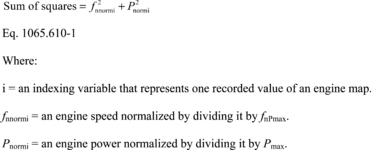(v) Determine the maximum value for the sum of the squares from the map and multiply that value by 0.98.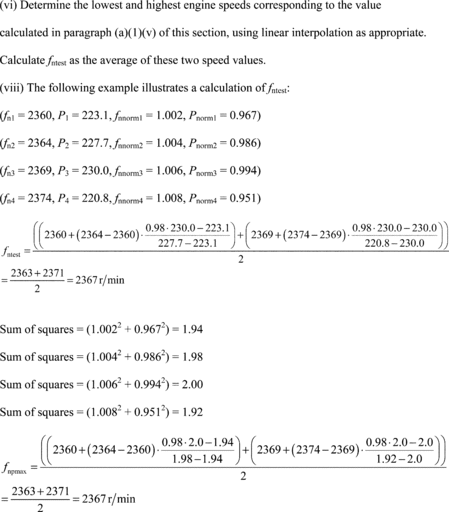(vi) Determine the lowest and highest engine speeds corresponding to the value calculated in paragraph (a)(1)(v) of this section, using linear interpolation as appropriate. Calculate fntest as the average of these two speed values. If there is only one speed corresponding to the value calculated in paragraph (a)(1)(v) of this section, take fntest as the speed where the maximum of the sum of the squares occurs.

(vii) The following example illustrates a calculation of fntest:

Pmax = 230.0

(fn1 = 2360, P1 = 222.5, fnnorm1 = 1.002, Pnorm1 = 0.9675) (fn2 = 2364, P2 = 226.8, fnnorm2 = 1.004, Pnorm2 = 0.9859) (fn3 = 2369, P3 = 228.6, fnnorm3 = 1.006, Pnorm3 = 0.9940) (fn4 = 2374, P4 = 218.7, fnnorm4 = 1.008, Pnorm4 = 0.9508) Sum of squares = (1.002 2 + 0.9675 2) = 1.94 Sum of squares = (1.004 2 + 0.9859 2) = 1.98 Sum of squares = (1.006 2 + 0.9940 2) = 2.00 Sum of squares = (1.008 2 + 0.9508 2) = 1.92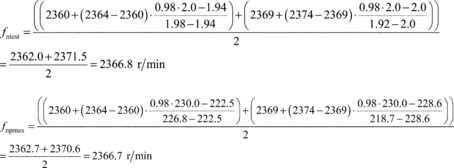(2) For engines with a high-speed governor that will be subject to a reference duty cycle that specifies normalized speeds greater than 100%, calculate an alternate maximum test speed, fntest,alt, as specified in this paragraph (a)(2). If fntest,alt is less than the measured maximum test speed, fntest, determined in paragraph (a)(1) of this section, replace fntest with fntest,alt. In this case, fntest,alt becomes the “maximum test speed” for that engine. Note that § 1065.510 allows you to apply an optional declared maximum test speed to the final measured maximum test speed determined as an outcome of the comparison between fntest, and fntest,alt in this paragraph (a)(2). Determine fntest,alt as follows: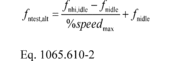Where: fntest,alt = alternate maximum test speed fnhi,idle = warm high-idle speed fnidle = warm idle speed % speedmax = maximum normalized speed from duty cycle Example: fnhi,idle = 2200 r/min fnidle = 800 r/min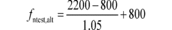fntest,alt = 2133 r/min

(3) For variable-speed engines, transform normalized speeds to reference speeds according to paragraph (c) of this section by using the measured maximum test speed determined according to paragraphs (a)(1) and (2) of this section - or use your declared maximum test speed, as allowed in § 1065.510.

(4) For constant-speed engines, transform normalized speeds to reference speeds according to paragraph (c) of this section by using the measured no-load governed speed - or use your declared maximum test speed, as allowed in § 1065.510.

(b) Maximum test torque, Ttest. For constant-speed engines, determine the measured Ttest from the torque and power-versus-speed maps, generated according to § 1065.510, as follows:

(1) For constant speed engines mapped using the methods in § 1065.510(d)(5)(i) or (ii), determine Ttest as follows:

(i) Determine maximum power, Pmax, from the engine map generated according to § 1065.510 and calculate the value for power equal to 98% of Pmax.

(ii) Determine the lowest and highest engine speeds corresponding to 98% of Pmax, using linear interpolation, and no extrapolation, as appropriate.

(iii) Determine the engine speed corresponding to maximum power, fnPmax, by calculating the average of the two speed values from paragraph (a)(1)(ii) of this section. If there is only one speed where power is equal to 98% of Pmax, take fnPmax as the speed at which Pmax occurs.

(iv) Transform the map into a normalized power-versus-speed map by dividing power terms by Pmax and dividing speed terms by fnPmax. Use Eq. 1065.610-1 to calculate a quantity representing the sum of squares from the normalized map.

(v) Determine the maximum value for the sum of the squares from the map and multiply that value by 0.98.

(vi) Determine the lowest and highest engine speeds corresponding to the value calculated in paragraph (a)(1)(v) of this section, using linear interpolation as appropriate. Calculate fntest as the average of these two speed values. If there is only one speed corresponding to the value calculated in paragraph (a)(1)(v) of this section, take fntest as the speed where the maximum of the sum of the squares occurs.

(vii) The measured Ttest is the mapped torque at fntest.

(2) For constant-speed engines using the two-point mapping method in § 1065.510(d)(5)(iii), you may follow paragraph (a)(1) of this section to determine the measured Ttest, or you may use the measured torque of the second point as the measured Ttest directly.

(3) Transform normalized torques to reference torques according to paragraph (d) of this section by using the measured maximum test torque determined according to paragraph (b)(1) of this section - or use your declared maximum test torque, as allowed in § 1065.510.

(c) Generating reference speed values from normalized duty cycle speeds. Transform normalized speed values to reference values as follows:

(1) % speed. If your normalized duty cycle specifies % speed values, use your warm idle speed and your maximum test speed to transform the duty cycle, as follows: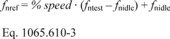Example: % speed = 85% = 0.85 fntest = 2364 r/min fnidle = 650 r/min fnref = 0.85 • (2364−650) + 650 fnref = 2107 r/min

(2) A, B, and C speeds. If your normalized duty cycle specifies speeds as A, B, or C values, use your power-versus-speed curve to determine the lowest speed below maximum power at which 50% of maximum power occurs. Denote this value as nlo. Take nlo to be warm idle speed if all power points at speeds below the maximum power speed are higher than 50% of maximum power. Also determine the highest speed above maximum power at which 70% of maximum power occurs. Denote this value as nhi. If all power points at speeds above the maximum power speed are higher than 70% of maximum power, take nhi to be the declared maximum safe engine speed or the declared maximum representative engine speed, whichever is lower. Use nhi and nlo to calculate reference values for A, B, or C speeds as follows: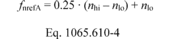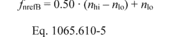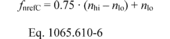Example: nlo = 1005 r/min nhi = 2385 r/min fnrefA = 0.25 • (2385−1005) + 1005 fnrefB = 0.50 • (2385−1005) + 1005 fnrefC = 0.75 • (2385−1005) + 1005 fnrefA = 1350 r/min fnrefB = 1695 r/min fnrefC = 2040 r/min

(3) Intermediate speed. Based on the map, determine maximum torque, Tmax, and the corresponding speed, fnTmax, calculated as the average of the lowest and highest speeds at which torque is equal to 98% of Tmax. Use linear interpolation between points to determine the speeds where torque is equal to 98% of Tmax. Identify your reference intermediate speed as one of the following values:

(i) fnTmax if it is between (60 and 75) % of maximum test speed.

(ii) 60% of maximum test speed if fnTmax is less than 60% of maximum test speed.

(iii) 75% of maximum test speed if fnTmax is greater than 75% of maximum test speed.

(d) Generating reference torques from normalized duty-cycle torques. Transform normalized torques to reference torques using your map of maximum torque versus speed.

(1) Reference torque for variable-speed engines. For a given speed point, multiply the corresponding % torque by the maximum torque at that speed, according to your map. If your engine is subject to a reference duty cycle that specifies negative torque values (i.e., engine motoring), use negative torque for those motoring points (i.e., the motoring torque). If you map negative torque as allowed under § 1065.510 (c)(2) and the low-speed governor activates, resulting in positive torques, you may replace those positive motoring mapped torques with negative values between zero and the largest negative motoring torque. For both maximum and motoring torque maps, linearly interpolate mapped torque values to determine torque between mapped speeds. If the reference speed is below the minimum mapped speed (i.e., 95% of idle speed or 95% of lowest required speed, whichever is higher), use the mapped torque at the minimum mapped speed as the reference torque. The result is the reference torque for each speed point.

(2) Reference torque for constant-speed engines. Multiply a % torque value by your maximum test torque. The result is the reference torque for each point.

(3) Required deviations. We require the following deviations for variable-speed engines intended primarily for propulsion of a vehicle with an automatic transmission where that engine is subject to a transient duty cycle with idle operation. These deviations are intended to produce a more representative transient duty cycle for these applications. For steady-state duty cycles or transient duty cycles with no idle operation, these requirements do not apply. Idle points for steady state duty cycles of such engines are to be run at conditions simulating neutral or park on the transmission.

(i) Zero-percent speed is the warm idle speed measured according to § 1065.510(b)(6) with CITT applied, i.e., measured warm idle speed in drive.

(ii) If the cycle begins with a set of contiguous idle points (zero-percent speed, and zero-percent torque), leave the reference torques set to zero for this initial contiguous idle segment. This is to represent free idle operation with the transmission in neutral or park at the start of the transient duty cycle, after the engine is started. If the initial idle segment is longer than 24 seconds, change the reference torques for the remaining idle points in the initial contiguous idle segment to CITT (i.e., change idle points corresponding to 25 seconds to the end of the initial idle segment to CITT). This is to represent shifting the transmission to drive.

(iii) For all other idle points, change the reference torque to CITT. This is to represent the transmission operating in drive.

(iv) If the engine is intended primarily for automatic transmissions with a Neutral-When-Stationary feature that automatically shifts the transmission to neutral after the vehicle is stopped for a designated time and automatically shifts back to drive when the operator increases demand (i.e., pushes the accelerator pedal), change the reference torque back to zero for idle points in drive after the designated time.

(v) For all points with normalized speed at or below zero percent and reference torque from zero to CITT, set the reference torque to CITT. This is to provide smoother torque references below idle speed.

(vi) For motoring points, make no changes.

(vii) For consecutive points with reference torques from zero to CITT that immediately follow idle points, change their reference torques to CITT. This is to provide smooth torque transition out of idle operation. This does not apply if the Neutral-When-Stationary feature is used and the transmission has shifted to neutral.

(viii) For consecutive points with reference torque from zero to CITT that immediately precede idle points, change their reference torques to CITT. This is to provide smooth torque transition into idle operation.

(4) Permissible deviations for any engine. If your engine does not operate below a certain minimum torque under normal in-use conditions, you may use a declared minimum torque as the reference value instead of any value denormalized to be less than the declared value. For example, if your engine is connected to a hydrostatic transmission and it has a minimum torque even when all the driven hydraulic actuators and motors are stationary and the engine is at idle, then you may use this declared minimum torque as a reference torque value instead of any reference torque value generated under paragraph (d)(1) or (2) of this section that is between zero and this declared minimum torque.

(e) Generating reference power values from normalized duty cycle powers. Transform normalized power values to reference speed and power values using your map of maximum power versus speed.

(1) First transform normalized speed values into reference speed values. For a given speed point, multiply the corresponding % power by the mapped power at maximum test speed, fntest, unless specified otherwise by the standard-setting part. The result is the reference power for each speed point, Pref. Convert these reference powers to corresponding torques for operator demand and dynamometer control and for duty cycle validation per 1065.514. Use the reference speed associated with each reference power point for this conversion. As with cycles specified with % torque, linearly interpolate between these reference torque values generated from cycles with % power.

(2) Permissible deviations for any engine. If your engine does not operate below a certain power under normal in-use conditions, you may use a declared minimum power as the reference value instead of any value denormalized to be less than the declared value. For example, if your engine is directly connected to a propeller, it may have a minimum power called idle power. In this case, you may use this declared minimum power as a reference power value instead of any reference power value generated per paragraph (e)(1) of this section that is from zero to this declared minimum power.

[73 FR 37324, June 30, 2008, as amended at 73 FR 59330, Oct. 8, 2008; 75 FR 23045, Apr. 30, 2010; 76 FR 57453, Sept. 15, 2011; 78 FR 36398, June 17, 2013; 79 FR 23783, Apr. 28, 2014; 80 FR 9118, Feb. 19, 2015; 81 FR 74170, Oct. 25, 2016]